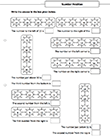## Ordinal number

##### 1. Ordinal number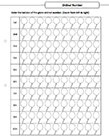##### 2. Ordinal number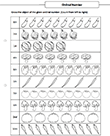##### 3. Ordinal number names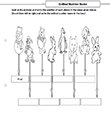##### 4. Ordinal number names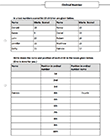##### 5. Ordinal number names##### 6. Ordinal number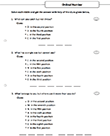##### 7. Ordinal number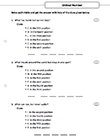##### 8. Number position